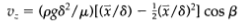Create an Account

Home / Questions / Different choice of coordinates for the falling film problem Re derive the velocity profil...

Different choice of coordinates for the falling film problem Re derive the velocity profile and the average velocity in S22 by replacing x by a coordinate x measured away from the wall that is

Different choice of coordinates for the falling film problem, Re-derive the velocity profile and the average velocity in S2.2, by replacing x by a coordinate x measured away from the wall; that is, x = 0 is the wall surface, and x = 8 is the liquid-gas interface. Show that the velocity distribution is then given by and then use this to get the average velocity. Show how one can get Eq. 2B.1-1 from Eq. 2.2-18 by making a change ofvariable.Jul 25 2020 View more View LessSubscribe To Get Solution